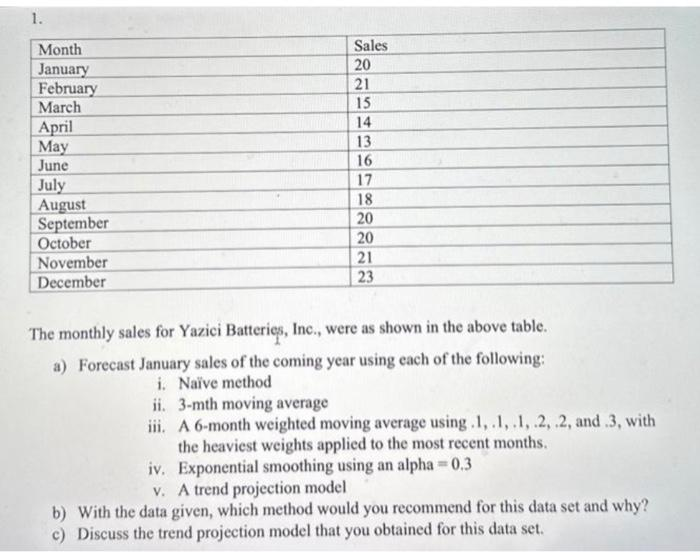# Question please show all work on excel  The monthly sales for Yazici Batteries, Inc., were as shown in the above table. a) Forecast January sales of the coming year using each of the following: i. Naive method ii. 3-mth moving average iii. A 6-month weighted moving average using .1, .1, .1, .2, .2, and .3, with the heaviest weights applied to the most recent months. iv. Exponential smoothing using an alpha $$=0.3$$ v. A trend projection model b) With the data given, which method would you recommend for this data set and why? c) Discuss the trend projection model that you obtained for this data set.Transcribed Image Text: The monthly sales for Yazici Batteries, Inc., were as shown in the above table. a) Forecast January sales of the coming year using each of the following: i. Naive method ii. 3-mth moving average iii. A 6-month weighted moving average using .1, .1, .1, .2, .2, and .3, with the heaviest weights applied to the most recent months. iv. Exponential smoothing using an alpha $$=0.3$$ v. A trend projection model b) With the data given, which method would you recommend for this data set and why? c) Discuss the trend projection model that you obtained for this data set.
Transcribed Image Text: The monthly sales for Yazici Batteries, Inc., were as shown in the above table. a) Forecast January sales of the coming year using each of the following: i. Naive method ii. 3-mth moving average iii. A 6-month weighted moving average using .1, .1, .1, .2, .2, and .3, with the heaviest weights applied to the most recent months. iv. Exponential smoothing using an alpha $$=0.3$$ v. A trend projection model b) With the data given, which method would you recommend for this data set and why? c) Discuss the trend projection model that you obtained for this data set.